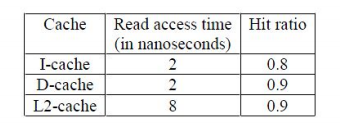# GATE | GATE-CS-2017 (Set 2) | Question 37

The read access times and the hit ratios for different caches in a memory hierarchy are as given below:The read access time of main memory in 90 nanoseconds. Assume that the caches use the referred-word-first read policy and the writeback policy. Assume that all the caches are direct mapped caches. Assume that the dirty bit is always 0 for all the blocks in the caches. In execution of a program, 60% od memory reads are for instruction fetch and 40% are for memory operand fetch. The average read access time in nanoseconds (up to 2 decimal places) is _________

Note: This question appeared as Numerical Answer Type.

(A) 2.74
(B) 4.72
(C) 3.10
(D) 2.67

Explanation: Since, L2 cache is shared between Instruction and Data.

Average Instruction fetch Time = L1 access time + L1 miss rate * L2 access time + L1 miss rate * L2 miss rate * Memory access time

= 2 + 0.2 * 8 + 0.2 * 0.1 * 90
= 5.4 ns

Average Data fetch Time = L1 access time + L1 miss rate * L2 access time + L1 miss rate * L2 miss rate * Memory access time

= 2 + 0.1 * 8 + 0.1 * 0.1 * 90
= 3.7 ns

= Fraction of Instruction Fetch * Average Instruction fetch time + Fraction of Data Fetch * Average Data Fetch Time

= 0.6×5.4+0.4×3.7 = 4.72ns

Option (B) is correct.

Quiz of this Question

My Personal Notes arrow_drop_up

Article Tags :

Be the First to upvote.

Please write to us at contribute@geeksforgeeks.org to report any issue with the above content.